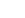# Basic JavaScript Loops

It often happens that you have to execute code blocks multiple times. You can do this effectively using JavaScript loops.

## For loops

This loop usually executes a command a certain number of times. You use statements to define the amount of times the command is executed. For loops have the following base-syntax:

``````for(initialization/start-value; condition; command){
//code block
}
```
```

As you can see, the for loop contains 3 parameters that are used to define how often the code block is executed:

• ### Initialization/counting variable

With this parameter you define a counting variable and its start-value. Multiple variables have to be separated by commas.

• ### Condition

The for loop is executed as long as this condition is true.

• ### Command

This command is executed every time after the execution of the code block.

The following code writes Toolinfy 5 times.

``````for(i=0; i<5; i++){
document.write("Toolinfy <br/>");
}
```
```

This code transformed into an actual sentence would be something like: "As long as `i` is smaller than 5 write Toolinfy and increment `i` by 1."

The counting variable can also be used in the code block. In the following example we'll multiply all numbers from 0 to 10 by 10 and show the results.

The following code writes Toolinfy 5 times.

``````for(i=0; i<=10; i++){
var timesten = i*10;
document.write(i + " x " + "10" + " = " + timesten + "*<br/>");
}
``````

This is how it would look:

## Do-while loops

Of course we don't always know how often we want to execute a command/code block. Instead we want execute this block until a certain condition isn't true anymore. The syntax of do-while loops looks like this:

``````do{
//code block
} while(condition);
``````

The following do-while loop will decrement the variable `example` by 1 as long as its bigger than 0.

``````var example = 10;
do{
example -= 1;
document.write(example + "<br/>");
} while(example > 0);
``````

Note that do-while loops are always executed at least once! This could lead to unwanted results.

## While loops

While loops basically work the same way that do-while loops do. The only difference is that do-while loops are executed once and then the condition is checked, which means that they are always executed at least once. The condition in a while loop is checked before it's executed. If the condition isn't fulfilled the code block won't be executed at all.